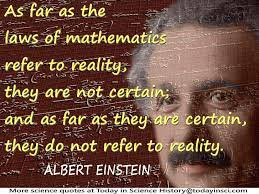# The Realty behind the wave function and Relativity

One can define reality as the world or the state of things as they actually exist, as opposed to an idealistic or notional idea of them.

Currently there are two ways science attempts to explain and define the reality of our universe. The first is Quantum mechanics or the branch of physics defines its evolution in terms of the probabilities associated with the wave function. The other is the deterministic environment of Relativity which defines it in terms of a physical interaction between space and time.

Specifically, Relativity would define the observable positions of particles in terms of where the point defining their center of mass is located.

While quantum mechanics uses the mathematical interpretation of the wave function to define the most probable position of a particle when observed.Since we all live in the same world you would expect the probabilistic approach of quantum mechanics to be compatible with the deterministic one of Einstein. Unfortunately, they define two different worlds which appear to be incompatible. One defines existence in terms of the probabilities while the other defines it in terms of the deterministic of properties of space and time.

However, to show why those probabilities appear to be incompatible with Relativity’s determinism even though they are NOT it will be necessary to explain the evolution of quantum environment in terms of a deterministic interaction between the components of a space-time environment.

For example, when we role dice in a casino most of us realize the probability of a six appearing is related to or is caused by its physical interaction with properties of the table in the casino where it is rolled. Putting it another way what defines the fact that six appears is NOT the probability of getting one but the interaction of the dice with the table and the casino it occupies.

This suggests to show the “reality” behind the wave function one MUST explain how its environment evolves in terms of how the physical components of space-time interact to define a particles position.

The fact that Relativity defines evolution of space-time in terms of the energy propagated by electromagnetic wave while Quantum Mechanics defines it in terms of the mathematical evolution of the wave function give us a starting point. This is because it suggests the evolution in both is defined in define by a wave.

To define the position of a particle in terms of the deterministic properties of Relativity one can use the science of wave mechanics along with the fact Relativity tells us an electromagnetic wave move continuously through space-time unless it is prevented from doing so by someone observing or something interacting with it. This would result in its energy being confined to three-dimensional space. The science of wave mechanic also tells us the three-dimensional “walls” of this confinement will result in its energy being reflected back on itself thereby creating a resonant or standing wave in three-dimensional space. This would cause its wave energy to COLLAPSE and be concentrated at the point in space were a particle would be found. Additionally, wave mechanics also tells us the energy of a resonant system, such as a standing wave can only take on the discrete or quantized values associated with its fundamental or a harmonic of its fundamental frequency. This means a particle would occupy an extended volume of space defined by the wavelength of its standing wave.

Putting it another way what defines the fact that a particle appears where it does is NOT determined by the probabilities associated with the wave function but a deterministic interaction of an electromagnetic wave with the physical properties of space-time.

(NOTE We will use a particles position to make the connection between the probabilities of Quantum mechanics and the determinism of Relativity but the same logic will apply to all conjugate pairs.)

However, the probabilistic interpretation of the wave function is defines its reality because it use a mathematical point to represent a position of a particle which it randomly places with respect to the center of a particle. Therefore, the randomness of where that point is with respect to a particle’s center will result in its position, when observed to be randomly distributed in space. This means one must define its position in terms of probabilities to average the deviations that are caused by that random placement.

Yet as was mentioned earlier Reality defines the position of particles in terms of where the point defining their center of mass is located. Therefore, because similar to quantum mechanics Relativity cannot precisely determine where that point is located it would also have to define their exact position in terms of probabilities.

However, the large number of particles in objects such as a moon or planet would result in averaging out the deviation of the position of each their individual particles it appears to be deterministic.

But the same logic would apply to a quantum environment because its probabilistic deviations of a particle’s position would average out making the position of large objects such as the mom and planets appear to be deterministic.

This suggests the reason our universe appears indeterminate on a quantum scale while being deterministic on a macroscopic level is because similar to Relativity those deviations would be averaged out by the large number of particles in objects like the moon and planets.

As was mentioned earlier one can define reality as the world or the state of things as they actually exist, as opposed to an idealistic or notional idea of them.

Therefore, as was shown above one can define the Reality of the probabilistic world of quantum mechanics and the deterministic one of Relativity by assuming actual existence of an electromagnetic wave whose evolution can be defined by the notional idea of the wave function.